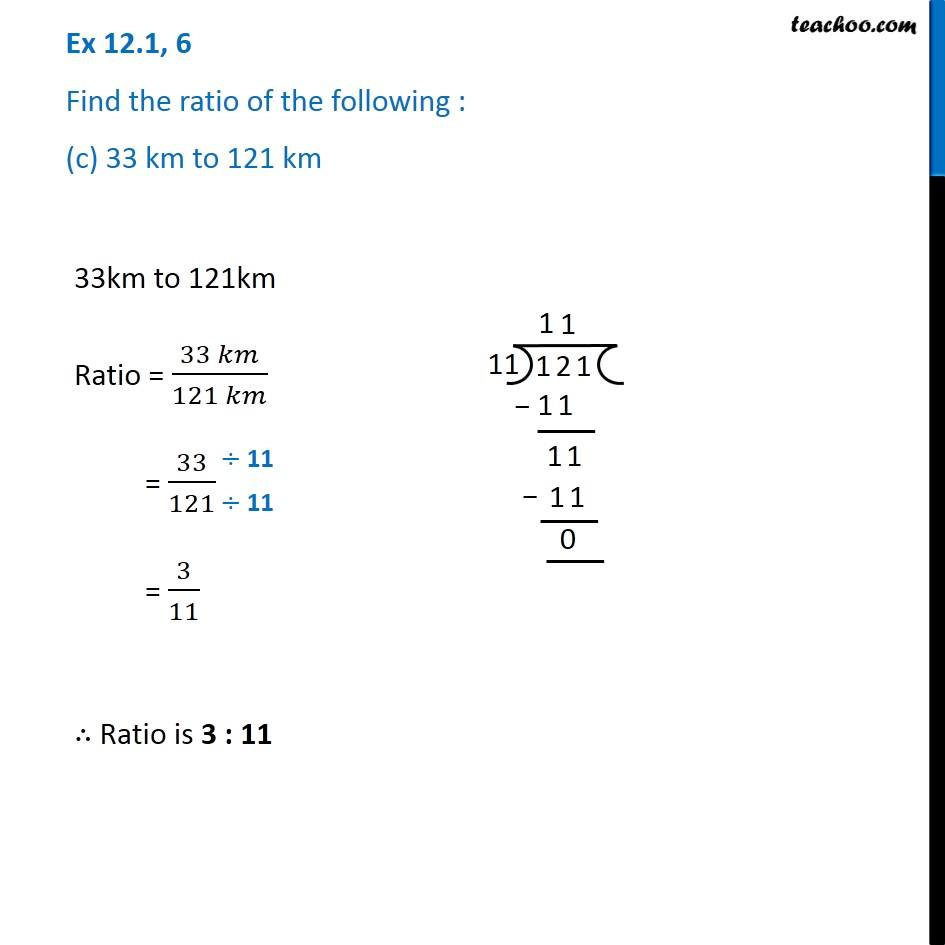1. Chapter 12 Class 6 Ratio And Proportion
2. Serial order wise
3. Ex 12.1

Transcript

Ex 12.1, 6 Find the ratio of the following : (c) 33 km to 121 km 33km to 121km Ratio = (33 𝑘𝑚)/(121 𝑘𝑚) = 33/121 = 3/11 ∴ Ratio is 3 : 11